• matlab训练svm模型
2021-04-29 10:20:09

clc;

clear;

close all;

traindata = [0 1; -1 0; 2 2; 3 3; -2 -1;-4.5 -4; 2 -1; -1 -3];

group = [1 1 -1 -1 1 1 -1 -1]';

testdata = [5 2;3 1;-4 -3];

svm_struct = svmtrain(traindata,group,'Showplot',true); % training

Group = svmclassify(svm_struct,testdata,'Showplot',true);

hold on;

plot(testdata(:,1),testdata(:,2),'ro','MarkerSize',12); % testing

hold off

程序运行结果如下：用大圈圈起来的是测试图像的结果，小圈圈起来的是支持向量。traindata中每行代表一个训练样本，group表示traindata中每个样本所属种类(只能有两个类)。

更多相关内容
• 各位朋友，labview如何调用MATLAB训练好的SVM模型呢?matlab
• 基于MATLABSVM支持向量机的数据分类，包括训练，测试，以及数据库 %第一步： %为了简化计算，可对得到的图像特征数据进行标准化 %将数据通过函数变换映射到[0.1,1] %第二步： %图像特征集合中信息特征的筛选：...
• mo_3.m_lbp特征提取，fitcecoc训练svm模型，predict预测，人脸分类。使用fitcecoc函数训练一个多分类的SVM模型，使用predict函数利用训练出的模型对测试数据进行预测，将得到的类标预测值与测试数据真实的类标进行...
• 目录 1.软件版本 ...MATLAB2021a 2.核心代码 function [model] = svmTrain(X, Y, C, kernelFunction, ... tol, max_passes) if ~exist('tol', 'var') || isempty(tol) tol = 1e-3; end if ~e

目录

1.软件版本

2.核心代码

3.操作步骤与仿真结论

4.参考文献

5.完整源码获得方式

MATLAB2021a

# 2.核心代码

function [model] = svmTrain(X, Y, C, kernelFunction, ...
tol, max_passes)

if ~exist('tol', 'var') || isempty(tol)
tol = 1e-3;
end

if ~exist('max_passes', 'var') || isempty(max_passes)
max_passes = 5;
end

% Data parameters
m = size(X, 1);
n = size(X, 2);

% Map 0 to -1
Y(Y==0) = -1;

% Variables
alphas = zeros(m, 1);
b = 0;
E = zeros(m, 1);
passes = 0;
eta = 0;
L = 0;
H = 0;

% Pre-compute the Kernel Matrix since our dataset is small
% (in practice, optimized SVM packages that handle large datasets
%  gracefully will _not_ do this)
%
% We have implemented optimized vectorized version of the Kernels here so
% that the svm training will run faster.
if strcmp(func2str(kernelFunction), 'linearKernel')
% Vectorized computation for the Linear Kernel
% This is equivalent to computing the kernel on every pair of examples
K = X*X';
elseif strfind(func2str(kernelFunction), 'gaussianKernel')
% Vectorized RBF Kernel
% This is equivalent to computing the kernel on every pair of examples
X2 = sum(X.^2, 2);
K = bsxfun(@plus, X2, bsxfun(@plus, X2', - 2 * (X * X')));
K = kernelFunction(1, 0) .^ K;
else
% Pre-compute the Kernel Matrix
% The following can be slow due to the lack of vectorization
K = zeros(m);
for i = 1:m
for j = i:m
K(i,j) = kernelFunction(X(i,:)', X(j,:)');
K(j,i) = K(i,j); %the matrix is symmetric
end
end
end

% Train
fprintf('\nTraining ...');
dots = 12;
while passes < max_passes,

num_changed_alphas = 0;
for i = 1:m,

% Calculate Ei = f(x(i)) - y(i) using (2).
% E(i) = b + sum (X(i, :) * (repmat(alphas.*Y,1,n).*X)') - Y(i);
E(i) = b + sum (alphas.*Y.*K(:,i)) - Y(i);

if ((Y(i)*E(i) < -tol && alphas(i) < C) || (Y(i)*E(i) > tol && alphas(i) > 0)),

% In practice, there are many heuristics one can use to select
% the i and j. In this simplified code, we select them randomly.
j = ceil(m * rand());
while j == i,  % Make sure i \neq j
j = ceil(m * rand());
end

% Calculate Ej = f(x(j)) - y(j) using (2).
E(j) = b + sum (alphas.*Y.*K(:,j)) - Y(j);

% Save old alphas
alpha_i_old = alphas(i);
alpha_j_old = alphas(j);

% Compute L and H by (10) or (11).
if (Y(i) == Y(j)),
L = max(0, alphas(j) + alphas(i) - C);
H = min(C, alphas(j) + alphas(i));
else
L = max(0, alphas(j) - alphas(i));
H = min(C, C + alphas(j) - alphas(i));
end

if (L == H),
% continue to next i.
continue;
end

% Compute eta by (14).
eta = 2 * K(i,j) - K(i,i) - K(j,j);
if (eta >= 0),
% continue to next i.
continue;
end

% Compute and clip new value for alpha j using (12) and (15).
alphas(j) = alphas(j) - (Y(j) * (E(i) - E(j))) / eta;

% Clip
alphas(j) = min (H, alphas(j));
alphas(j) = max (L, alphas(j));

% Check if change in alpha is significant
if (abs(alphas(j) - alpha_j_old) < tol),
% continue to next i.
% replace anyway
alphas(j) = alpha_j_old;
continue;
end

% Determine value for alpha i using (16).
alphas(i) = alphas(i) + Y(i)*Y(j)*(alpha_j_old - alphas(j));

% Compute b1 and b2 using (17) and (18) respectively.
b1 = b - E(i) ...
- Y(i) * (alphas(i) - alpha_i_old) *  K(i,j)' ...
- Y(j) * (alphas(j) - alpha_j_old) *  K(i,j)';
b2 = b - E(j) ...
- Y(i) * (alphas(i) - alpha_i_old) *  K(i,j)' ...
- Y(j) * (alphas(j) - alpha_j_old) *  K(j,j)';

% Compute b by (19).
if (0 < alphas(i) && alphas(i) < C),
b = b1;
elseif (0 < alphas(j) && alphas(j) < C),
b = b2;
else
b = (b1+b2)/2;
end

num_changed_alphas = num_changed_alphas + 1;

end

end

if (num_changed_alphas == 0),
passes = passes + 1;
else
passes = 0;
end

fprintf('.');
dots = dots + 1;
if dots > 78
dots = 0;
fprintf('\n');
end
if exist('OCTAVE_VERSION')
fflush(stdout);
end
end
fprintf(' Done! \n\n');

% Save the model
idx = alphas > 0;
model.X= X(idx,:);
model.y= Y(idx);
model.kernelFunction = kernelFunction;
model.b= b;
model.alphas= alphas(idx);
model.w = ((alphas.*Y)'*X)';

end


# 3.操作步骤与仿真结论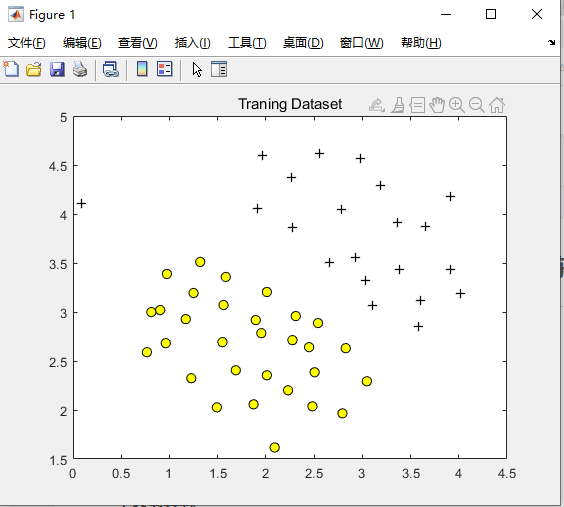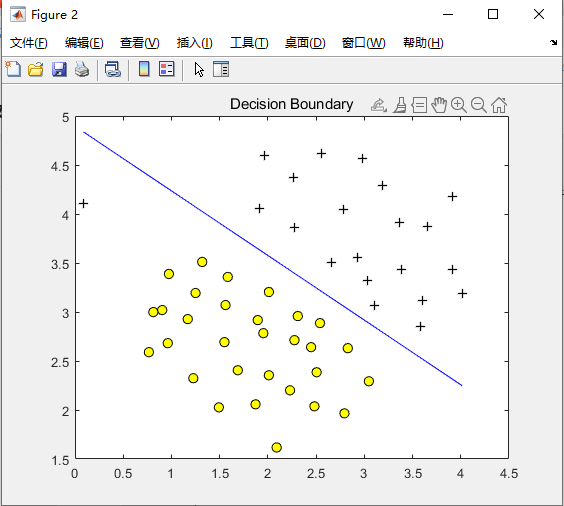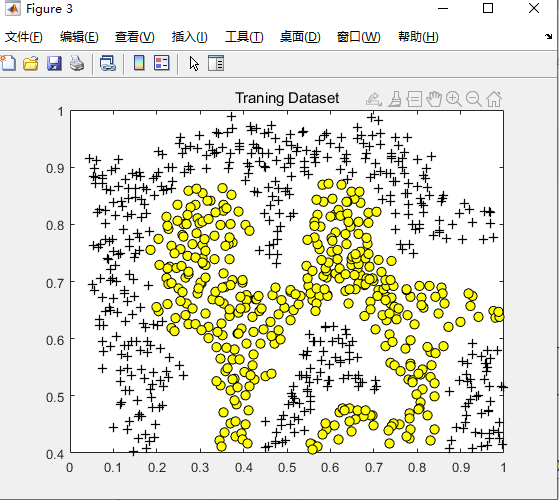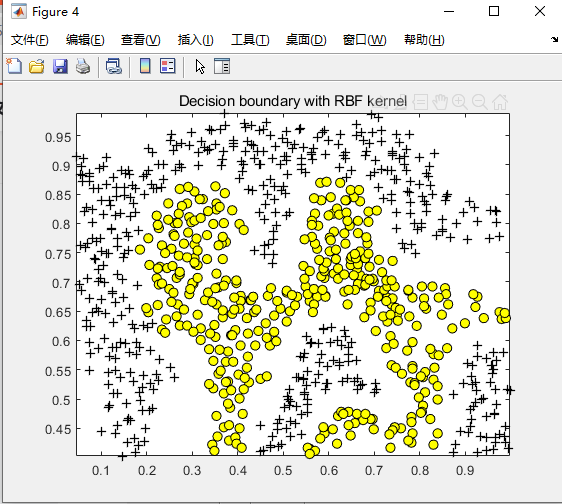# 4.参考文献

 Osuna E ,  Freund R ,  Girosi F . Training svm: An application to face detection.  1997.

D224

# 5.完整源码获得方式

方式1：微信或者QQ联系博主

展开全文SVM
• 搜索了好多文章，matlab自带的svm工具保存训练好的模型，读取离线模型的数据少之又少，libsvm倒是有一点，但是之前的代码会用自带工具箱做的，又懒得换。那就自己搞吧！首先上训练函数的代码：flow_svmstruct = ...

搜索了好多文章，matlab自带的svm工具保存训练好的模型，读取离线模型的数据少之又少，libsvm倒是有一点，但是之前的代码会用自带工具箱做的，又懒得换。那就自己搞吧！

首先上训练函数的代码：

flow_svmstruct = svmtrain(flow_traindata,flow_group,'Kernel_Function','RBF') % training

训练好的模型保存在flow_svmstruct结构体中，结构体里面的内容如下：

flow_svmstruct =

SupportVectors: [8x1 double]

Alpha: [8x1 double]

Bias: 0.3798

KernelFunction: @rbf_kernel

KernelFunctionArgs: {}

GroupNames: [64x1 double]

SupportVectorIndices: [8x1 double]

ScaleData: [1x1 struct]

FigureHandles: []

所以，我们要做的工作是：先将这个结构体保存下来，然后分类的时候再读取出来。

1.如何保存？

如何保存结构体呢？经过一番调研，可以用save命令把结构体保存成矩阵(.mat)的形式,具体代码如下：

执行完这一行代码，model文件夹下多了一个文件：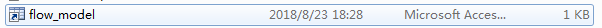说明保存成功了。

2.如何读取？

但是，我们最终想要的结果是将flow_svmstruct这个结构体提取出来，经过调试发现，flow_model_mat这个矩阵大小是1行1列，内容是flow_svmstruct，所以经过以下操作就可以完美地提取出来保存的数据了

flow_svmstruct = flow_model_mat.flow_svmstruct;

小结：

%训练并保存数据

flow_svmstruct = svmtrain(flow_traindata,flow_group,'Kernel_Function','RBF'); % training

%读取保存的模型数据并分类

flow_svmstruct = flow_model_mat.flow_svmstruct;

G1= svmclassify(flow_svmstruct,flow_testdata);

展开全文• 流程：整理训练网络的数据，对样本进行处理 -> 建立卷积神经网络-> 将数据代入进行训练 -> 保存训练好的模型（从全连接层提取特征） -> 把数据代入模型获得特征向量 -> 用特征向量代替原本的输入送入SVM训练 -> 测试...
• 支持向量机的模型训练源代码，平台为C++，可用于回归和分类问题
• 随着人工智能的崛起，MATLAB也添加了自己的机器学习工具包，只需要很少的代码或命令就能完成模型训练和测试的过程，训练好的模型也能方便的导出，供VC等调用。本文主要介绍模型训练、导出和调用的整个过程。 软件...

MATLAB是美国MathWorks公司出品的商业数学软件，为算法开发、数据可视化、数据分析以及数值计算等提供了高级计算语言和交互式环境。随着人工智能的崛起，MATLAB也添加了自己的机器学习工具包，只需要很少的代码或命令就能完成模型训练和测试的过程，训练好的模型也能方便的导出，供VC等调用。本文主要介绍模型训练、导出和调用的整个过程。

软件版本：

VC2015，matlab2018a

1，数据准备。

随机生成一组训练数据，格式如下：

x,y,label

x,y为坐标，label为标签

我生成的数据总量为3000，格式如下：

前1500：x>0, y>0,   label=1      x，y落在坐标系的第一象限

后500  ：x<0, y>0,   label=0      x，y落在坐标系的第二象限

后500  ：x<0, y<0,   label=0      x，y落在坐标系的第三象限

后500  ：x>0, y<0,   label=0      x，y落在坐标系的第四象限

数据存为csv格式，如下图所示：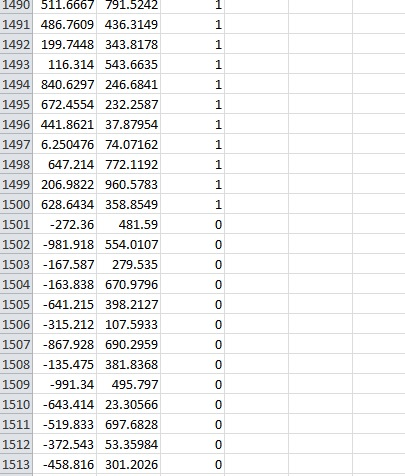2，模型训练

（1）打开matlab，将工作目录设置在cvs文件所在目录，点开APPS选项卡，点击Classification Learner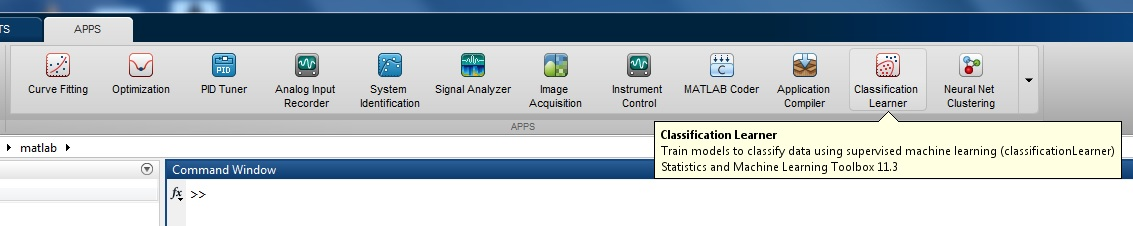（2）点击菜单：New Session，选择From File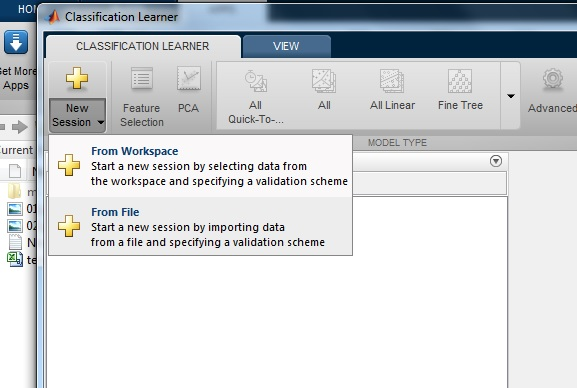（3）选择我们生成的训练数据文件后，matlab显示出了文件内容，点击Insert Selection导入文件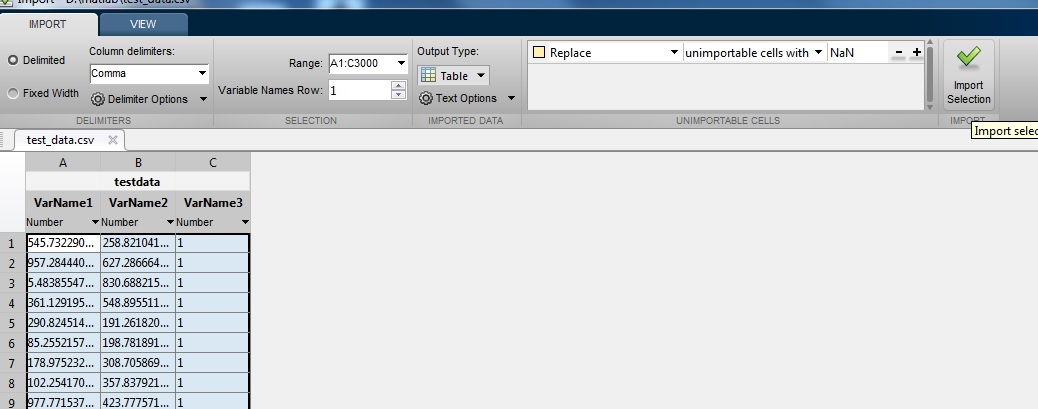（4）数据设置，前两列为训练数据，第三列为标签，点击Start Session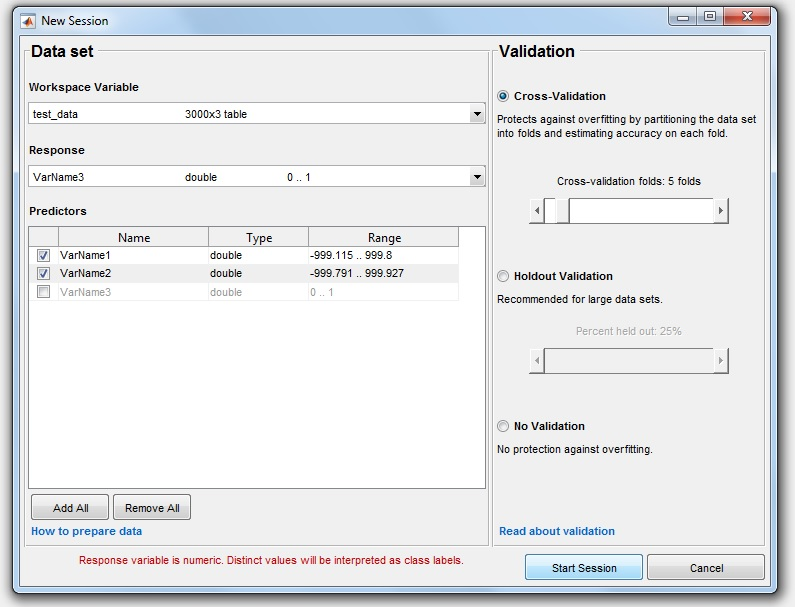数据导入后，分类器显示数据点的分布情况，可以看到：标签为1的样本显示为红色，分布在第一象限；标签为0的样本显示为蓝色，分布在二三四象限。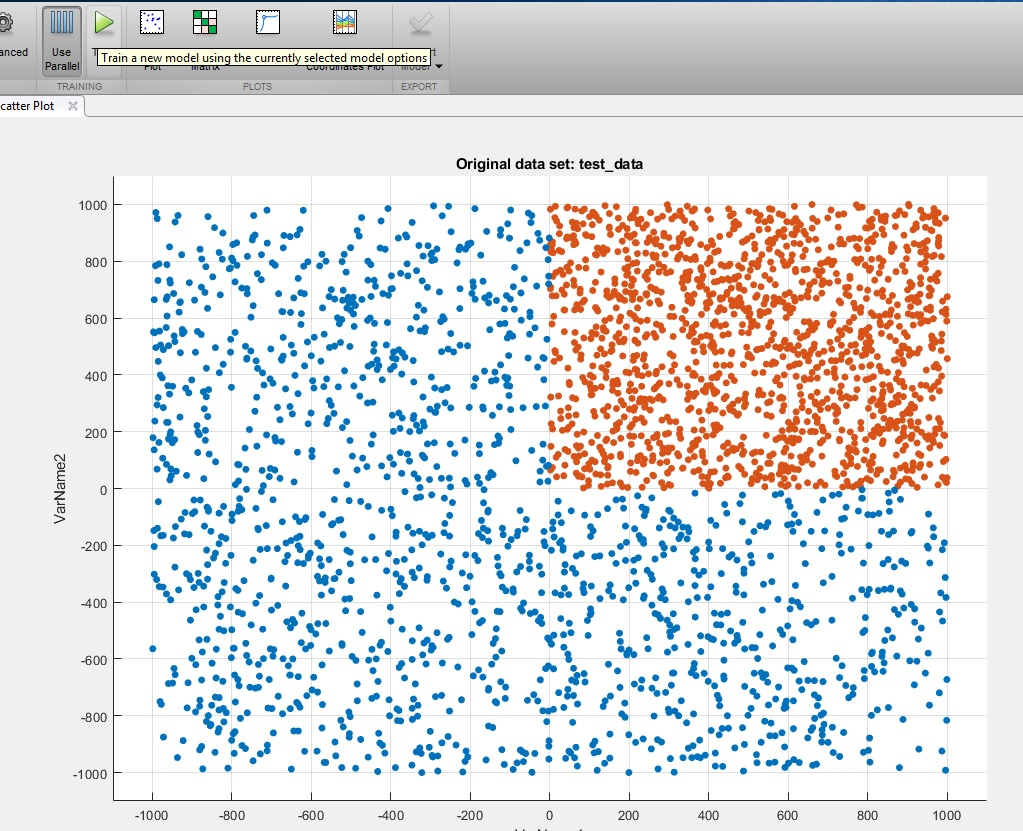（5）开始训练，此时分类器默认选择的模型为Fine Tree，我们可以直接使用这个模型进行训练，也可以选择ALL，让所有的模型都进行训练，从而根据训练结果选择最好的模型，这里我们直接点击Train进行训练。训练完成后显示Fine Tree模型对于当前样本的识别率为100%（matlab将导入的样本分为两份，一份训练，一份测试，100%是对测试样本集测试后得出的结果）。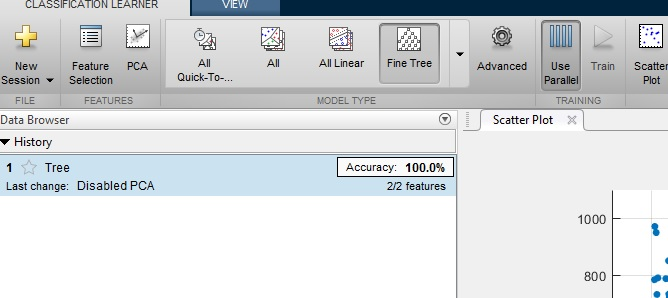3，模型导出及使用

选择训练好的Fine Tree模型，点击Export Model，选择Export compact model，导出训练好的模型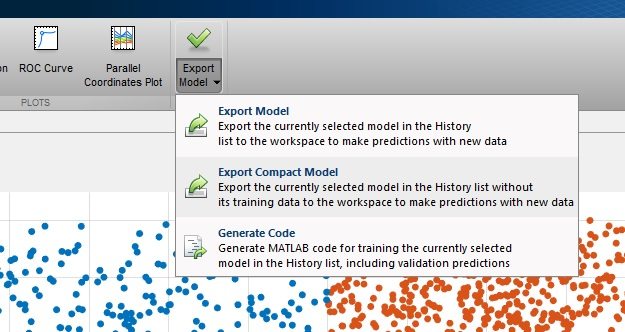模型导出后，在matlab的变量列表里，出现了导出的模型：Trained model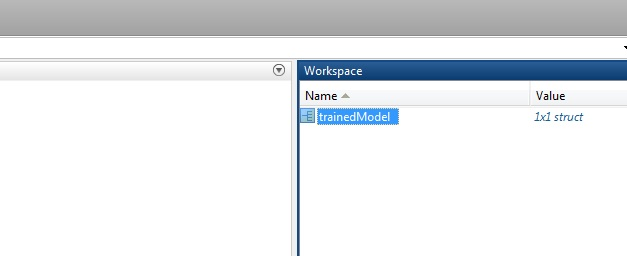双击Trained model，可以看到模型的内部结构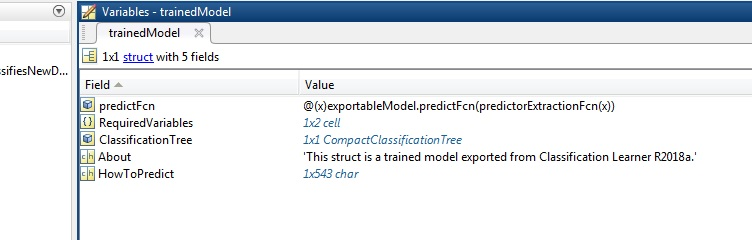使用命令，将模型里的分类器导出为mat文件：

saveCompactModel(trainedModel.ClassificationTree, 'ClassificationTree')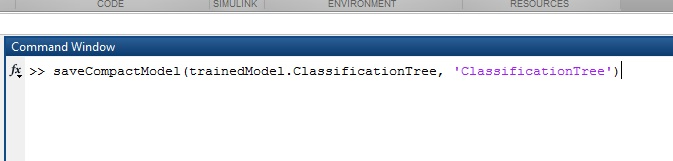命令执行成功后，工作目录出现ClassificationTree.mat文件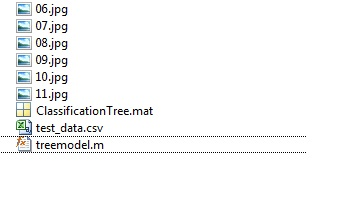此mat文件无法被VC直接使用，可以在matlab里封装一个函数，函数里使用此分类器进行分类，然后将此函数导出C/C++代码或dll供VC使用。

function label = treemodel(input)
label = predict(model,input);
end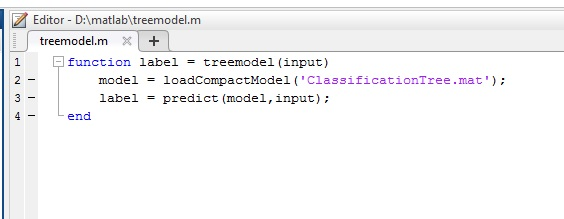在matlab命令行里输入coder，打开代码转换工具，将封装的matlab函数导出为C/C++代码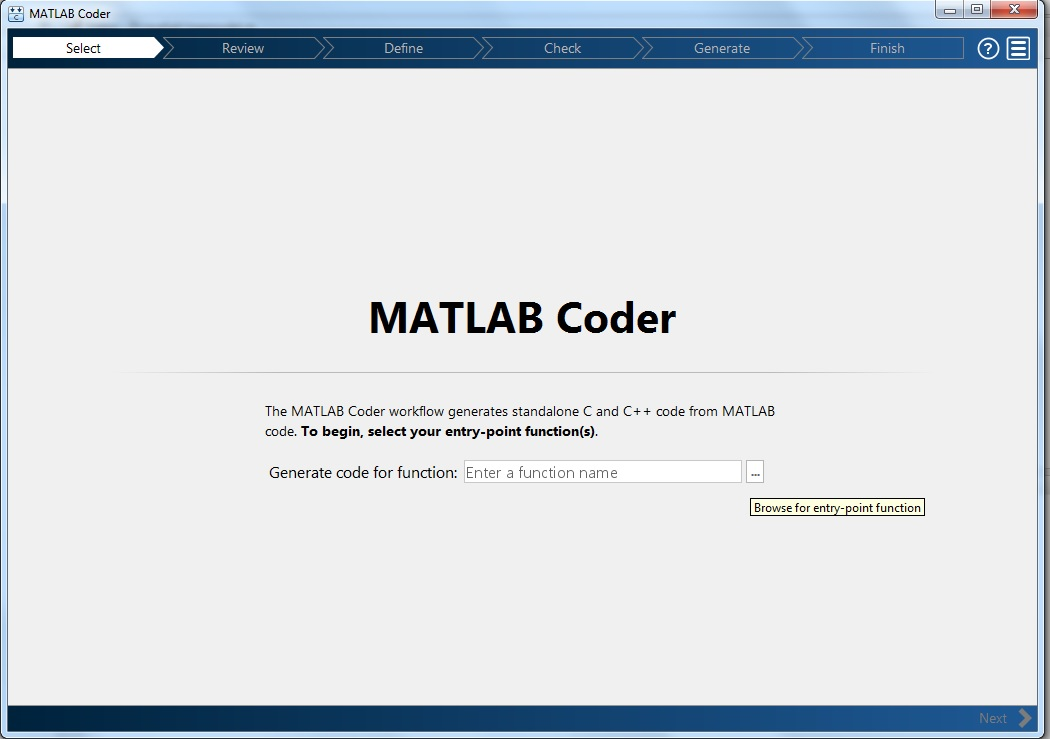点击...选择刚才封装好的.m文件， 点击下一步，进入输入参数定义界面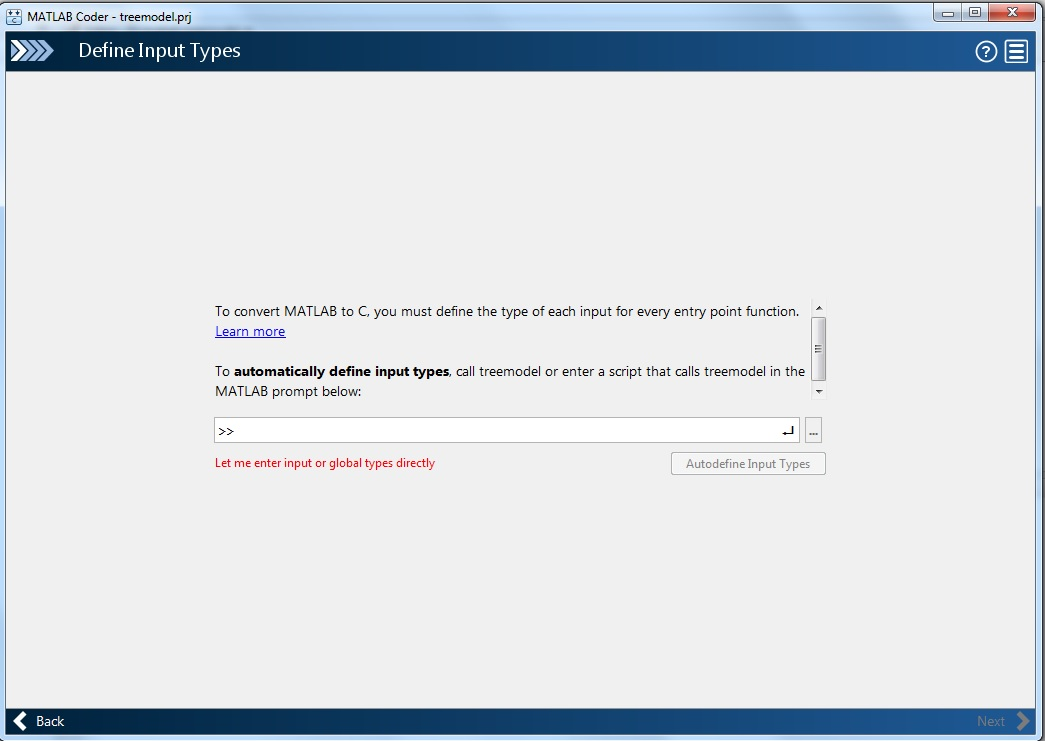点击let me enter input or global types directly，定义输入参数：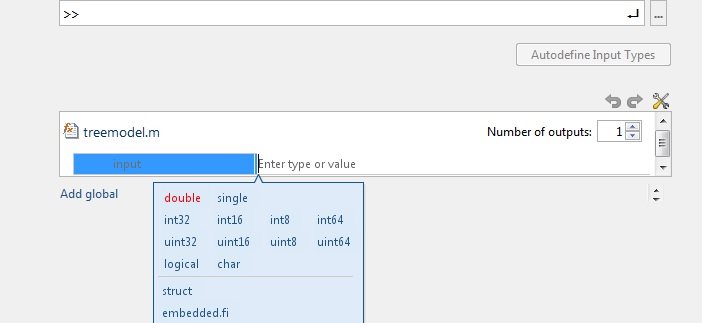输入类型为double类型的x，y坐标，所以选择double，类型为1*2（选择1xn，n的位置输入2）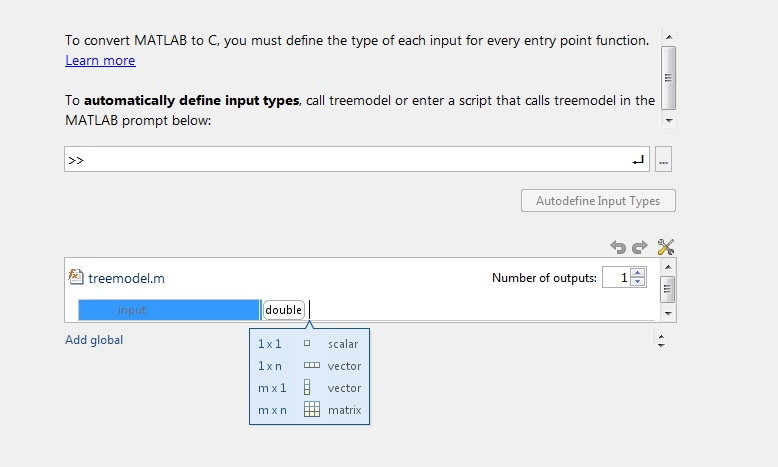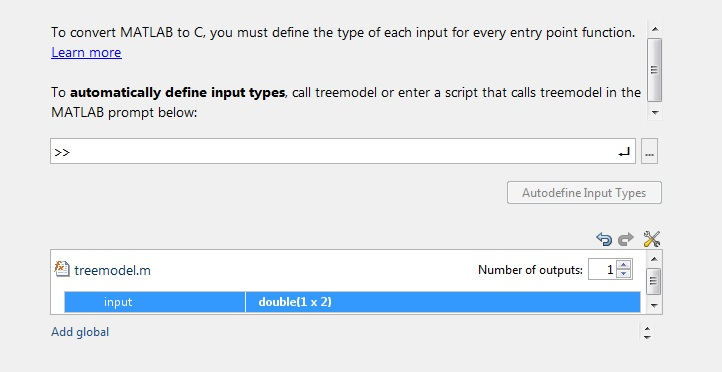选择下一步，点击Generate生成代码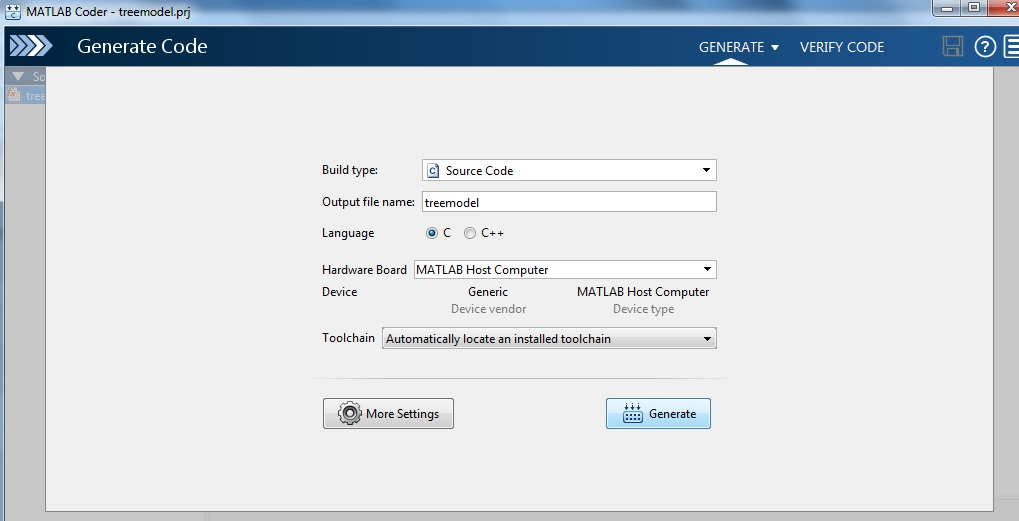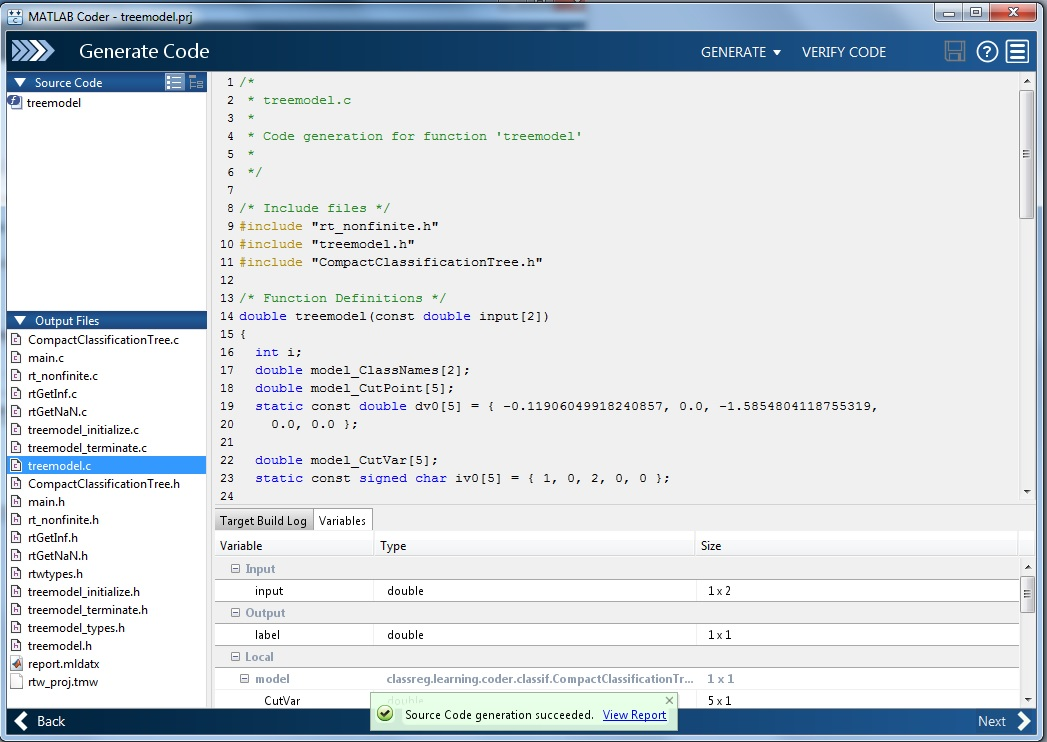生成成功后，matlab工作目录出现codegen文件夹，里边是生成的文件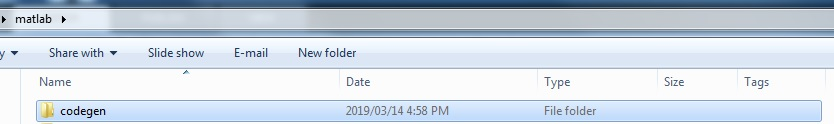将图中所有的.c和.h文件导入VC工程（.c文件去掉预编译头选项），然后打开examples文件夹，查看示例文件main.h和main.c，仿照其示例调用分类函数进行分类测试（注意：头文件包含使用extern "C"方式）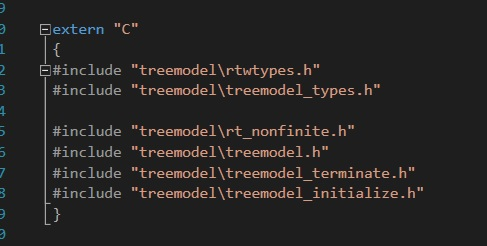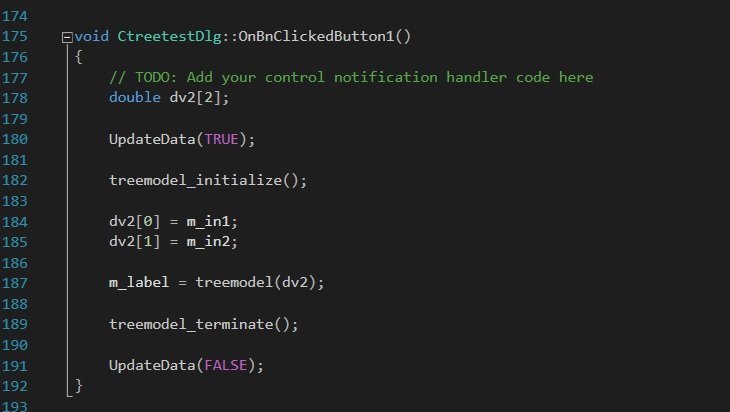最后一步，输入任意数据进行测试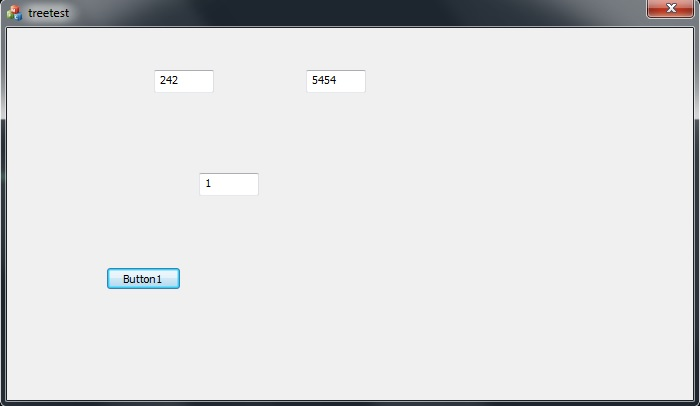至此，所有流程全部完成。

展开全文matlab训练模型 matlab导出模型
• 使用SVM模型的分数计算标准ROC曲线。 在同一样本数据上拟合朴素贝叶斯分类器。 计算后验概率（分数） [~,score_nb] = resubPredict(mdlNB); 使用朴素贝叶斯分类的分数计算标准ROC曲线。 将ROC曲线绘制在同一张图上...Matlab SVM 朴素贝叶斯模型 分类
• 将“CrossVal”设置为“on”并将训练后的模型提取到crossval()函数都旨在实现同样的目标.你可以使用其中一种,这取决于你.kFoldLoss()是一个函数本身,不包含在“CrossVal”标志中.它将交叉验证的模型作为输入.无论您...
• 步骤2：训练SVM模型 使用生成的CSV文件，将它们放置在DATA文件夹中。 它们将用于创建SVM模型。 genFullModelBySegments.m 当处理512个样本的批次减少了其创建较少特征的样本总数时，此代码被创建为工件去除（使用...
• SVM算法：Support Vector Machine (SVM，支持向量机) 是一种监督学习方法，能最小化经验误差和最大化几何边缘，被称为最大间隔分类器，可以用于分类与回归分析。最早的相关论文是美国贝尔实验室的Corinna Cortes和...
• 使用matlab实现SVM做线性分类、非线性分类以及模型参数自动优化。支持向量机 matlab 机器学习
• hog svm matlab代码此文件夹用于存放好的/坏的锅过滤器代码，如果您想...三、运行hog_svm.py，使用hog_svm模型进行训练和测试； 或者您可以运行 train.py 和 test.py 以使用基于 tensorflow 的 ML 模型进行训练和测试。
• matlab 使用svm进行分类含实例代码（适用于二分类和多分类matlab 支持向量机 matlab svm svm
• 支持向量机进行预测SVMMatlab版） 使用支持向量机进行预测。调用示例： in=load('testData.txt'); SVM(in(:,2:12),in(:,1),3)支持向量机
• svm算法手写matlab代码Matlab机器学习工具集 该代码包含用MATLAB编写的机器学习算法和演示，并用于完成Stanfords机器学习课程。 工作总结： 表示我完成的程序功能。 示例1：假设您是大学部门的管理员，并且您想根据...
• 传统的SVM做的事情其实就是找到一个超平面，实现二分类，一类+1，一类-1。如上所示。它的目的就是使得两类的间隔最大。只要确定了间隔最大和两边距离相等，就能确定唯一平面。黑色的块表示距离分割面最近的样本向量...matlab svm 机器学习
• ## SVM回归预测模型

千次阅读 2021-04-24 19:25:28
我把14章，SVM用于回归建模的例子改动后，结果出现了问题：首先，我描述一下，我想做的东西：我要预测输出的压力，当前的输出的压力和前3个时刻的5个变量有关，我也想减少变量，可是预测的效果就不好了)。...
• 最近一直在用matlab和libsvm，发现libsvm库用起来还是很方便的，就是没有模型直接保存到文件和读取模型matlab接口(C++的接口有)。由于有会用的Opencv等C/C++库，所以数据交换比较麻烦。看了一下libsvm的svm.h、svm...
• 由于刚接触SVM，用的程序是网上下载的一个标准的演示程序,在该程序基础上改进的.请帮忙看看！谢谢！其中Calckernel函数是一般的核函数计算，没有错误，就不再贴这部分程序了。clear all% --------------------------...
• 1 模型 基于数据的机器学习就是由观测样本数据得出目前尚不能通过原理分析得到的规律,利用其对未来数据进行预测.神经网络以其优越的函数逼近性能广泛用于建立时间序列过去与未来数据之间某种确定的映射关系,实现...matlab 机器学习 神经网络
• 在文件svm_test.m中运行示例SVM_SMO 使用 SMO 求解器创建 SVM 模型矩阵 x(mxn) 包含 m 个测试和 n 个特征的训练集带有相应的标签向量 y(mx1)。 SMO 求解器用户常数 C, tol(tolerance and eps (epsilon). 内核的选择...matlab
• MATLAB小技巧（12）SVM简单实例前言一. MATLAB仿真一：线性可分二. MATLAB仿真二：非线性可分三. 小结 前言 MATLAB进行图像处理相关的学习是非常友好的，可以从零开始，对基础的图像处理都已经有了封装好的许多可...matlab 支持向量机 图像处理 线性分类 非线性分类
• 对于组合二元支持向量机模型的多类学习，使用纠错输出码（ECOC，error-correcting output codes ）。有关详细信息，请参阅fitcecoc。 ECOC 可以用来将 Multiclass Learning 问题转化为 Binary Classification 问题。...matlab SVM 多分类
• 基于SVM的疲劳驾驶系统。基于神经网络的非接触式疲劳驾驶检测已成为当前针对疲劳驾驶检测领域炙手可热的研究方向。它有效解决了接触式疲劳检测方法给驾驶员带来的干扰以及单一信号源对于反映疲劳程度可靠性低的问题...
• svmtrain(1,2,3,4,5,6)可见该训练模型函数有6个参数的得到训练model。1.第一个是需要训练的数据(a1,a2,a3...) ai=(x1,x2,x3...)(xi代表不同的属性值)2.第二个参数是训练数据的标记lambel，一般是-1和+1。注意的是第......